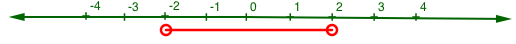### Home > INT1 > Chapter Ch11 > Lesson 11.2.1 > Problem11-53

11-53.

Solve the inequalities and equations below. Represent your solutions for each inequality on a number line.

1. $\left|x\right|+3<5$

Isolate the absolute value by subtracting $3$ from both sides.
$|x| + 3 < 5$
$|x| + 3 − 3 < 5 − 3$
$|x| < 2$

Change the inequality into an equation and solve for $x$.
$|x| < 2$
$|x| = 2$
$x = \text{{2, −2}}$

Graph both values of $x$ on the number line, which divides the line into three regions.
Substitute a value from each region into the original inequality.
Whichever regions make the inequality true are the solutions.1. $5(2x+1)\ge30$

Change to an equation and solve for $x$.
$5(2x + 1) ≥ 30$
$5(2x + 1) = 30$
$x = 2.5$

Graph $x$ on a number line.
Then check values from both regions to find the solution.

$x ≥ 2.5$
Don't forget to represent your solution with a number line!

1. $\frac { 1 } { x } - \frac { 5 } { 2 } = \frac { 3 } { 2 }$

Multiply both sides by $2x$ as Fraction Buster to eliminate the fractions.

$\left(\frac{1}{x}\right)2x-\left(\frac{5}{2}\right)2x=\left(\frac{3}{2}\right)2x$

$2 − 5x = 3x$

$x=\frac{1}{4}$

1. $−5−x>3−x$

Add x to both sides.
$−5 − x + x > 3 − x + x$
$−5 > 3$
Is this statement true? If so, then x can be any real number. If not, then there is no real solution.

1. $3\sqrt{4-x}+1=13$

Subtract $1$ from both sides. Then divide by three.
This will isolate the square root. Look inside to determine the solution.

1. $|x+1|\le4$

See the help for part (a).